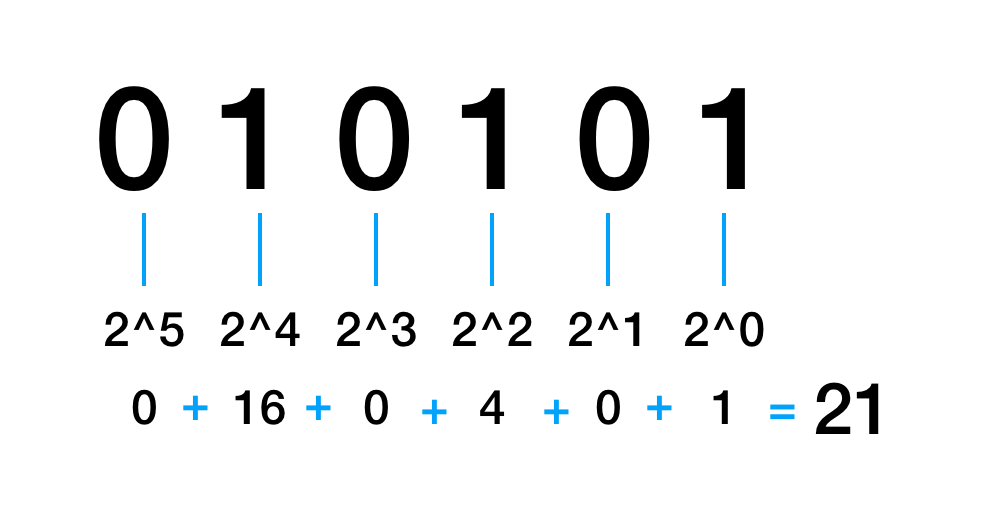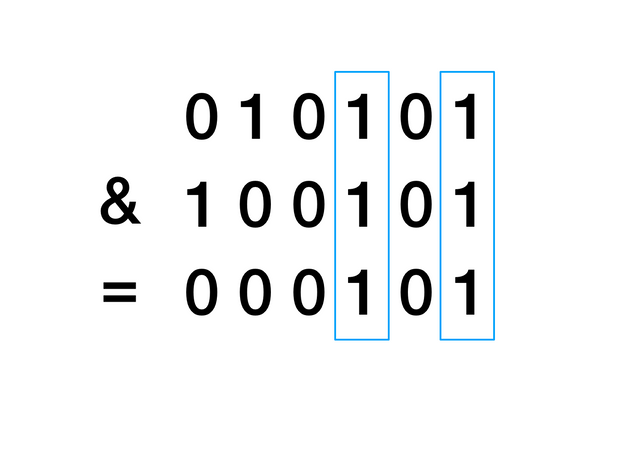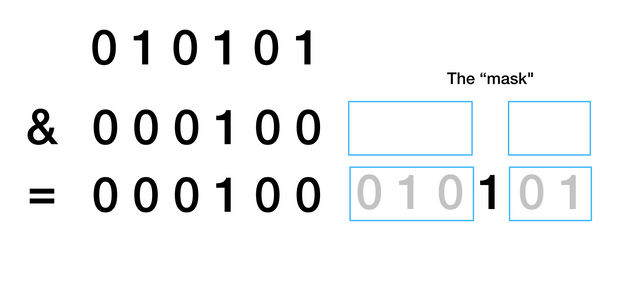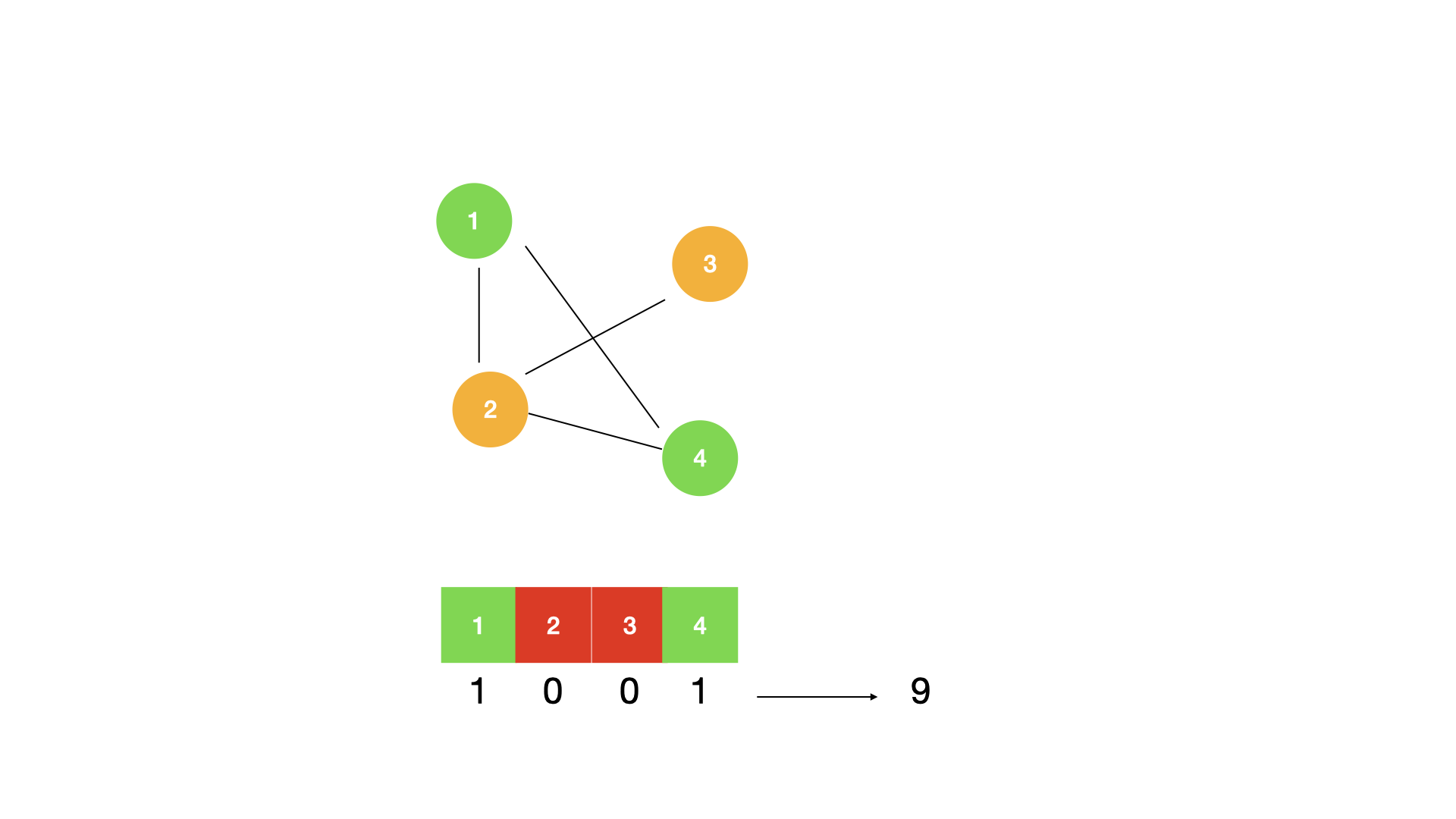### Binary Numbers

At the smallest scale in computers, data is stored as bits. A bit stores either 0 or 1. A binary number is a number expressed in the base-2 system. Each digit can be either 0 or 1. The integer types we use in programming languages are actually stored as binary numbers. Here is how binary numbers converts to decimal numbers:You can check that in python:

``````1>>> bin(21)
2'0b10101'``````

Note that `0b` means it's a binary number. The leading 0s are omitted and that's why we have `10101` instead of `010101`.

### Bitwise AND

We can `AND` two binary numbers by comparing each digit. If they are both 1 then the resulting digit is 1, otherwise it's 0.Now finally, what's a mask? We can construct a binary number such that a certain digit is set to 1 and other digits are all set to 0. This creates a "mask" that when we `AND` it to another binary it "turns off" (set to 0) all digits except the 1 digit in the mask.## Encoding the state

How is this all useful to algorithms and dynamic programming? The answer is to encode states. Remember in backtracking problems like permutation, we used a `used` boolean array to keep track of state, i.e. which character has been used. If we were to memoize the state, we need to somehow serialize the array, e.g. turning it into a string which has extra computational cost. With a bitmask, the serialization from boolean array to integer is already done for you - it's just an integer. This reduces memory consumption and makes the algorithm faster and simpler.

For instance, let us say that we are on a graph, and the problem requires us to remember all the nodes that we visited. We can encode each node as a boolean bit value as part of our bitmask and use that value as our dp state.In the example, we have visited node `1` and node `4`. We encode that as `1001` which is equal to `2^0 + 2^3 = 9`.

Bitmask questions can vary and additional dp states may be required so the code provided will loosely follow what a bitmask type question will look like.

## Common bit manipulation operations

Typically, these problems use a recursive function as well in order to do dp. Below is some pseudocode to outline this idea. Here are some useful operations to keep in mind:

1. `(1 << i)` -> simply to access the `i`th bit in the mask such as through a loop

2. `(bitmask | (1 << i))` -> this operation sets the `i`th bit in the bitmask to `1`

3. `(bitmask & ~(1 << i))` -> this operation sets the `i`th bit in the bitmask to `0`

4. `(bitmask & (1 << i))` -> this operation checks if the `i`th bit in the bitmask is set to `1` or not

Here's another example.

## Use bitmask to generate all subsets:

``````1nums = [1, 2, 3]
2n = len(nums)
3subsets = []
4for mask in range(2 ** n):
5    subset = []
6    for i in range(n):
7        if mask & (1 << i):
8            subset.append(nums[i])
9    subsets.append(subset[:])
10
11# subsets = [[], , , [1, 2], , [1, 3], [2, 3], [1, 2, 3]]``````

We didn't need to use backtracking for this. Pretty cool, eh!

Now here is pseudocode to show how to use bitmask for dynamic programming.

``````1function f(int bitmask, int [] dp) {
4    }
5    for each state you want to keep track of {
6        if current state not in mask {
Bitmask is helpful with problems that would normally require factorial complexity (something like `n!`) but can instead reduce the computational complexity to `2^n` by storing the dp state. It can also act as an effective "upper-bound" on the number of computations that can take place.